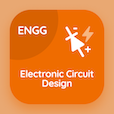Online Engineering Degree Courses

Electronic Circuit Design Quizzes

Electronic Circuit Design Quiz - Complete

Practice Diode Limiting and Clamping Circuits quiz questions and answers, diode limiting and clamping circuits MCQs with answers PDF, quiz 78 to learn Electronic Circuit Design online course. Diode applications MCQ questions, diode limiting and clamping circuits Multiple Choice Questions (MCQ Quiz) for online college degrees. Diode Limiting and Clamping Circuits Book PDF: mosfet, diode, decibel, transistor as an amplifier, diode limiting and clamping circuits test prep for online colleges enrolling.

"A device that inserts a positive DC level in the output voltage is called" MCQ PDF: diode limiting and clamping circuits App APK with diode limiter, diode blocker, diode clamper, and diode barrier choices for college entrance exams. Solve diode applications questions and answers to improve problem solving skills for top engineering universities.

## Trivia Quiz on Diode Limiting and Clamping Circuits MCQs

MCQ: A device that inserts a positive DC level in the output voltage is called

Diode blocker
Diode limiter
Diode Clamper
Diode barrier

MCQ: If the output voltage of the transistor amplifier is 4V RMS and the input is 100mV rms, the voltage gain will be

40
4
400
0.4

MCQ: Positive dBm values represent power levels

Above 1mW
Below 1mW
Equal to 0
Equal to infinity

MCQ: The two layers of positive and negative charges together form a

Depletion region
P-type region
N-type region
Pn region

MCQ: Mid point bias for a D-MOSFET is ID equals to

Zero
Infinite
IDSS
One

### Practice Quizzes from Electronic Devices Book